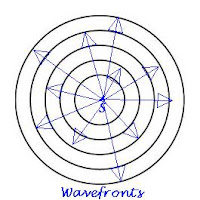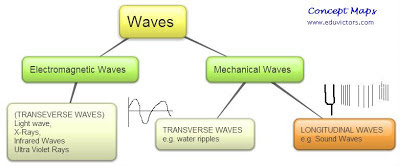## Friday, 25 January 2013

### CBSE Class 9 - Science - CH12 - Sound (Q and A)

SOUND

(Q & A)

Q1: What is Sound? Why it is important for us?

Answer: Sound is a longitudinal mechanical wave. Sound has great importance in our daily life. It gives us a sensation of hearing. It makes possible to communicate with other persons through speech.

Q2: What is a mechanical wave?

Answer: A mechanical wave is a disturbance that moves through a medium when the particles of the medium set neighbouring particles into motion. The particles vibrating in turn do not move forward but the disturbance is carried forward.

Q3: How does sound travel in gases and liquids as longitudinal or as transverse waves?

Q3: What is a longitudinal wave?

Answer: If the particles of a medium vibrate in a direction, parallel to or along the direction of propagation of wave, it is called longitudinal wave.

Q4: Give examples of longitudinal waves.Particles at the same distance are in the same phase and constitute a wave front. Concentric circles of sound wave here represent wavefronts.

Q5: What are wavefronts?

Answer: Wavefronts are the surfaces over which the vibrations due to sound wave have same value. For near sound source, wavefronts are considered spherical while for the distance source of sound the wavefronts are considered planar.

Q6(NCERT): Why sound waves are called mechanical waves?

Answer: Sound or Sound energy cannot be generated as its own. Some mechanical energy is required which make the body vibrate. Thus it is the mechanical energy gets converted to sound energy which travels through medium. Therefore sound waves are mechanical waves?

Q7: Can sound wave travel in vacuum?

Answer: No Sound is a mechanical wave, it requires a medium to travel.

Q8: What type of waves can travel in vacuum? Give example(s).

Answer: Electromagnetic waves can travel in vacuum. Sun light, X-rays are examples of electromagnetic waves.

Q9(NCERT): Suppose you and your friend are on the Moon. Will you be able to hear any sound produced by your friend?

Answer: No we will not hear any sound on the Moon. The Moon does not have any atmosphere, since sound is a mechanical wave and requires a medium to travel.

Q10: What is speed of sound in air?

Answer: At 0° C it is 331 m/s. At 20° celcius it is 341 m/s.

Q11: What are the factors speed of sound wave depends upon?

Answer: Speed of the sound depends on the following factors:
1. inertial property of the medium (to store kinetic energy)
2. elastic property of the medium (to store potential energy)
3. temperature of the medium
4. density of the medium
5. humidity present in the medium (in air/gases).

Q12: Among solids, liquids and gases sound travels faster in which medium?

Answer: Sound travels the fastest in solids.

Q13(CBSE 2010): Three persons, A, B and C are made to hear a sound travelling through different mediums as given below:

 Persons Medium A Iron Rod B Air C Water
Who will hear the sound first? Why?

Answer: Person A will hear the sound first because sound travels the faster in solids than in liquids and gases.

Q14(NCERT): How does the sound produced by a vibrating object in a medium reach your ear?

Answer: Sound is a mechanical longitudinal wave. It requires a medium e.g. air in which it travels in the form of rarefactions and compressions and reaches our ears.

Q15: What is the audible range?

Q16: What is a transverse wave?

Answer: A transverse wave is one in which the particles of the medium vibrate in a direction perpendicular to the direction of propagation of the wave.Heinrich Hertz - who studied about electro-magentic waves. Unit of frequency is named after him. image credits:wikimedia

Q17: Why transverse wave does not travel through air or gases?

Answer: In transverse waves, the particles of the medium vibrate perpendicular to the direction of propagation of the wave. The gas particles are loosely packed and compressible. The gas particles do not vibrate in perpendicular direction to the direction of propagation of sound.

Q18(NCERT): What are wavelength, frequency, time period and amplitude of a sound wave?

Wavelength(λ): The distance between two consecutive compressions or two consecutive
rarefactions is known as the wavelength. Its SI unit is metre (m).

Time period(T): Time taken by a particle of the medium to complete one vibration is called Time period. Its unit is second.

Frequency (ν): The number of  vibrations completed by a particle in one second is called frequency . Its unit is hertz. i.e. ν = 1/T.

Amplitude(a): The maximum displacement of a particle from the mean position is called amplitude. Its unit is metre.

Q19: What is a wave number?

Answer: The number of wavelengths present per unit length is called wave number. i.e. it is the reciprocal of wavelength = 1/λ.

Q20(NCERT): How are the wavelength and frequency of a sound wave related to its speed?

Answer: Speed (v) = Wavelength(λ) × frequency (ν)

Q21(CBSE 2011): A sound wave travels at a speed of 339 ms–1. If its wavelength is 1.5 cm, what is the frequency of the wave?

Answer: Given speed(v) = 339 ms–1. λ = 0.0015 m, ν = ?
Since v = λ × ν ⇒ ν = v/λ = 339/0.0015 = 22600Hz

Q22(NCERT): Calculate the wavelength of a sound wave whose frequency is 220 Hz and speed is
440 m/s in a given medium.

Answer: Given ν = 220Hz, speed(v) = 440 m/s  and λ = ?
Since v = λ × ν ⇒ λ = v/ν = 440/220 = 2m

Q23: Write differences between Transverse waves and Longitudinal waves.

S.No.Transverse WavesLongitudinal Waves
1Particles of medium vibrate  perpendicular to the direction of propagation of wave. Particles of medium vibrate along the direction of propagation.
2Waves are propagated in the form of crests and troughs.Waves are transmitted in the medium in the form of rarefactions and compressions.
3No pressure variation in the medium.Pressure and density of the medium are maximum at compressions and minimum at rarefactions.
4e.g. waves in solids, liquid surfacese.g. Travel in solids, liquids and gases.

Q24(CBSE 2011): A hospital uses an ultrasonic scanner to locate tumors in a tissue. What is the wavelength of sound in a tissue is which the speed of sound is 1.7 km/s. The operating frequency of the scanner is 4.2 MHz. (1MHz = 106 Hz).

Answer: Given ν = 4.2 MHz = 4.2 × 106 Hz,
speed(v) = 1.7 km/s= 1.7 × 103 m/s
and λ = ?
Since v = λ × ν ⇒ λ = v/ν = 1.7 × 103  / 4.2 × 106  = 0.405 × 10-3 m = 4.05 × 10-4 m

Q25(CBSE 2011): If 20 waves are produced per second, what is the frequency in hertz?

Answer: Number of waves per second is known as frequency. ∴ frequency(ν) = 20Hz

Q26(CBSE 2011): What is reverberation?

Answer:  The repeated reflection that results in the persistence of sound is called reverberation.

Q27: What is echo?

Answer: The sound waves produced bounce back or gets reflected from the mountain or buildings and come to our ears, this reflected sound is known as Echo. To hear echo, the barrier reflecting the sound should be least at a distance of 17 meters.

Q28: What is infrasonic? Give an example.

Answer: Sound having frequency less than 20Hz is known as infrasonic sound or infrasonic.
Waves produced during earthquake are infrasonic.

Q29: Give examples of organisms which can hear infrasonic?

Answer: Whales and elephants can produce and hear infrasonic sound.

Q30: What are ultrasonics?

Answer: Sound having frequency greater than 20,000 Hz is called ultrasonics or ultrasonics sound.
Animals like bat, cat and dogs are capable of hearing ultrasonics.

Q31: Give an application use of ultrasonics.

Answer: Ultrasonic sounds are used in medical field for diagnosis and treatment of internal illness of human body. e.g. kidney or gall bladder stones can be detected by ultrasound. Using ultrasound shock, such stone can be broken into small grains which later on get flushed out with urine.

Q32(CBSE 2011): What type of wave is represented by density- distance graph?

Q33(SQP): Which wave characteristics determine the
(a) Loudness

(b) pitch of sound?

Draw two different waveforms and mark these characteristics on it.

Answer:  (a) Loudness is determined by the amplitude of the sound wave. Higher the amplitude, higher will be the loudness.

(b) Frequency determines the pitch of the sound.

Q34(CBSE 2010): Demonstrate an activity to show that sound requires a medium to travel.

1. Take a bell jar and place an electric bell inside it.
2. Connect the bell to a battery.
3. Close the electric circuit by pressing the switch.
4. Light bulb glows indicates circuit is closed. Sound of the bell is heard due to presence of air in the bell jar.
5. Now evacuate  the air through evacuation pump.
6. Press the switch again. Light bulb glows but no sound is heard. It shows air medium is required for the sound wave to travel.

Q35(NCERT): Guess which sound has a higher pitch: guitar or car horn?

Answer: Pitch is directly proportional to the frequency of sound wave. Since the frequency of sound wave generated by a guitar is higher than that of card horn, pitch of the guita will be higher.

Q36(NCERT): A person is listening to a tone of 500 Hz sitting at a distance of 450 m from the source of the sound. What is the time interval between successive compressions from the source?

Answer: The time interval between successive compressions of a sound wave is equal to the timer period of the wave.  (Given Frequency = 500Hz)
Time Period (T) = 1/frequency
⇒ T = 1/500Hz = 0.002 s

Q37(NCERT): Distinguish between loudness and intensity of sound.

Num.Intensity of sound                        Loudness
1.The amount of sound energy passing each second through unit area is called the intensity of sound.Loudness is the amount of sound energy perceived by human ear.
2.This property depends on source of the sound.Loudness depends on the source, medium and the receiver.
3.It is a quantitative term. It is qualitative term.
4.It is measured as watt/metre2 i.e. energy per unit areait is measured in decibels (db)

Q38: A man stands in between two cliffs and fires a gun. He hears two successive echoes after 3s and 5s . What is the distance between two cliffs?
(speed of sound = 330 m/s).

Answer:  (Note: A man is standing between two cliffs)
Distance traveled by sound = Speed of Sound × time of echo/2

Distance (D1) from first Cliff (nearer one) = 330 × 3s/2 = 495 m
Distance (D2) from second Cliff (distant one) = 330 × 5s/2 = 825 m

Distance between the two cliffs = D2 + D1 = 825 + 495  = 1320m

.1.2.Really, It helped me a lot. Thanks.

3.4.5.Very well explained. Good information. Thank you.

1.not a very well its a best & its also a short point of full ch

6.very good please update more questions and also upload some study material videos

7.thank youso much

8.1.5 cm isn't equal to 0.0015 m but it is 0.015 m ...........am I right?????

1.yes 1.5 cm is 1.5/100 m

2.Yeah u r right

9.nice information provided... thanks

10.11.12.13.14.15.16.17.